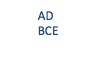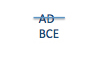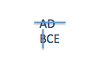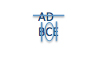# How Data Sufficiency Works

by , Jan 14, 2013Lets just put it right out there: data sufficiency is bizarre. If youre just starting out, youre probably thinking, What is this thing? Even if youve been studying for a while, unless you really like math, you probably feel a little uncomfortable whenever a DS question pops up on the screen.

Why? Because we all realize that we could completely mess up a DS question and still get to one of the 5 answer choices, clueless that weve messed up. Its not like Problem Solving, where at least I know when I mess up because my answer isnt in the answer choices! (Actually, a lot of the time, we still get an answer thats in the answer choices even on PS but we persist in feeling that PS is more straightforward because the answers are real.)

## What is DS?

The GMAT really isnt a math test. These tests are actually trying to test us on our executive reasoning skills that is, how well we make decisions and prioritize when faced with too many things to do in too short a length of time.

Data Sufficiency questions test our ability to (quickly) analyze a collective set of data and figure out which pieces are needed to do the job. Imagine your boss dumping a bunch of stuff on you and saying, Hey, our client wants to know whether they should raise the price on this product. Can you answer that question from this data? If so, which pieces do we need to prove the case?

We do, of course, have to do some math and sometimes that math is quite annoying. We usually dont, however, have to do as much as is necessary on the more normal quant questions (PS).

## How does DS work?

If you already feel comfortable with the basics of DS, you may want to skim or skip through this particular section of the article.

First, were given whats called the question stem. Heres an example:

How old is Oliver?

The question stem asks us a question, naturally. It can also provide information, such as:

If Olivers age is even, how old is Oliver?

Now we know that Olivers age is an even number. If they told me, for example, that Oliver is either 13 or 14 years old, I know he must be 14, since I can only consider even numbers as possible values for Olivers age.

Next, the problem will give us two statements, such as:

(1) Oliver is 4 years older than Sam.

(2) Sam will be 11 years old in 5 years.

So, can we figure out how old Oliver is? What information would we need to do so? The first statement, by itself, doesnt help, because we dont know how old Sam is. The second statement, by itself, also doesnt help, because it doesnt tell us anything about Oliver.

If we put the two statements together, however, then we can actually figure out how old Oliver is. In this case, using both statements 1 and 2 together is sufficient to answer the question. (And this situation corresponds to answer choice C on the GMAT.)

If you havent already, go and try the very first question in the Data Sufficiency section of The Official Guide 13th Edition (OG13). If you feel pretty comfortable with DS already, try question 44 instead.

Next, there are 5 possible answers to Data Sufficiency questions:

(A) Statement 1 does allow us to answer the question but statement 2 does not.

(B) Statement 2 does allow us to answer the question but statement 1 does not.

(C) Neither statement works on its own, but I can use them together to answer the question.

(D) Statement 1 works by itself and statement 2 works by itself.

(E) Nothing works. Even if I use both statements together, I still cant answer the question.

Heres an easier way to remember the five answer choices; we call this the twelve-ten mnemonic (memory aid):

1: only statement 1

2: only statement 2

T: together

E: either one

N: neither / nothing

## Value vs. Yes/No

It turns out that all DS questions can be put into one of two broad categories: value questions or yes/no questions. Our Oliver question, above, was an example of a value question: we were asked to find a specific value (Olivers age). If we can find one specific value, then that information is sufficient to answer the question. If, on the other hand, a statement gives us zero values or more than one value, then that statement is NOT sufficient.

Maybe half of our DS questions will be yes/no questions rather than value questions. Instead of asking how old Oliver is, the question might ask Is Oliver 13 years old? Lets say we discover that Oliver is actually in his twenties. Whats the answer to that question? No. Is that answer sufficient? Yes!

That, in a nutshell, illustrates the weirdness of yes/no questions. A definitive NO answer to a question is a sufficient answer. Do I know how old Oliver is? Nope, I only know hes in his twenties. But I wasnt asked how old he is. The question simply asked whether Oliver is 13, and I know the answer to that: definitively no. Therefore, the information is sufficient to provide a definitive answer to the question.

If, on the other hand, someone said, Oliver? Oh, hes either 13 or 22, now I would have a maybe, maybe not answer, or sometimes yes, sometimes no. Thats not sufficient to answer the question.

In short, a definitive yes answer is sufficient and a definitive no answer is also sufficient. On the other hand, sometimes yes and sometimes no is NOT sufficient. (And, of course, I dont know anything at all about this is also not sufficient.)

Want to try this out on a real yes/no question? If youre just getting started, try data sufficiency question 7 in OG13. If you feel more comfortable with yes/no already, try question 32.

Finally, just a note. All questions, whether value or yes/no, have this in common:

• a definitive answer to the question (no ambiguity) is sufficient; the answer is always 3 or always yes or always this one response
• an it might be this way or that way answer is NOT sufficient; the answer is 3 or 14; the answer is yes and no; the answer is it depends

## Whats my overall DS strategy?

A new question pops up on the screen. Now what?

First, read the question stem (this is everything above the two statements labeled 1 and 2). Write down any facts, formulas, or info that you want in writing. Note whether you have a value or yes/no question.

Next, glance very briefly at the two statements, just long enough to notice the way in which the information is presented. Written out or pure math? With real numbers? Variables? Percentages or fractions? Fairly simple / straightforward, or more complicated? Do NOT actually do anything with the statements at this point.

Now, go back to the question stem and ask yourself: what else can I figure out or infer from this information? Can I simplify anything? Can I rephrase anything? Unless the question stem is extremely simple, you can probably do something with that information right now that will make your life easier once you get to the statements. Simplify a complex formula. Combine two pieces of info to get a third piece. (Follow the link included in this paragraph to get more ideas about how to rephrase the given information.)

Okay, youre ready to tackle the statements. By default, youre going to start with statement 1. If you look at statement 1, however, and think, Yuck! (or, if you can see out of the corner of your eye that statement 2 is extremely simple), then start with statement 2 instead.

Lets start with statement 1 on this one. Write AD on your scrap paper. Below that, write BCE. Now, evaluate statement 1. Lets use our Oliver problem:

How old is Oliver?

(1) Oliver is 4 years older than Sam.

(2) Sam will be 11 years old in 5 years.If statement 1 IS sufficient, cross off the bottom row of letters (BCE). If statement 1 is NOT sufficient, cross off the top row of letters. In this case, statement 1 does not allow us to figure out how old Oliver is, so we cross off the top row.Next, evaluate statement 2. This is important: completely ignore everything contained in statement 1. Just look at statement 2 all by itself. In this case, statement 1 wasnt sufficient, so we have answers B, C, and E left. If statement 2 IS sufficient, then the answer is B. If statement 2 is NOT sufficient, cross off B and move to the final evaluation. In this case, statement 2 by itself tells us nothing about Olivers age, so cross off answer B.Side note: if statement 1 had been sufficient, wed have had answers A and D left. If statement 2 IS also sufficient, then the answer is D. If statement 2 is NOT sufficient, the answer is A. Okay, back to the Oliver problem.

The next step is to evaluate statements 1 and 2 together. (Note: If youve already found an answer, you dont have to do this; you only have to do this if you have crossed off answers A, B, and D, as weve done on this problem.)

If the two statements together ARE sufficient, the answer is C. If the two statements together are NOT sufficient, the answer is E. In this case, if Sam will be 11 years old in 5 years, then we know how old Sam is right now. Since we also know how much older Oliver is than Sam, we can figure out Olivers age. Statements 1 and 2 together ARE sufficient, so the answer is C.I want to point out one important thing. Did you notice that I didnt actually calculate either Sams age or Olivers age? That wouldnt have been difficult to do and yet I avoided doing it. Its important to build this habit on DS: only calculate when you have to calculate. If you know that you can calculate something, though, then thats enough and we dont have adequate time on this test to bother calculating numbers that we dont actually need!

What if we had started with statement 2 first? The process is actually almost exactly the same, but we have to make one change at the start. Instead of using the AD/BCE answer grid, we instead use the BD/ACE answer grid (that is, we swap A and B in the answer grid). Other than that, everything works exactly the same.

Go ahead and try the problem again, only this time use BD/ACE and start with the second statement.

## What else do I need to know?

An enormous amount, actually. This article barely scratches the surface of DS. There are all kinds of great strategies out there how to test numbers, how to prove insufficiency, how to use theory vs. real numbers, and so on. If youre taking a class or using some kind of a test-prep book, then you should be getting this strategy as part of your regular program. If youre not, then you should make sure to seek out Data Sufficiency strategies during your preparation; such strategies will change the game for you!

Meanwhile, here are a couple of example problems to get you started:

GMATPrep Inequalities

GMATPrep Divisibility

Happy studying!